# Demand curve

View other price-quantity curves

## Definition

The demand curve for a good is defined with the following in the background:

• The specific good.
• A unit for measuring the quantity of that.
• A unit for measuring price.
• A convention on whether sales taxes are included in the stated price.
• A certain set of economic actors who are the potential buyers of that good.
• A time frame within which the demand is measured.
• An economic backdrop that includes all the determinants of demand other than the unit price of that good.

The demand curve has the following characteristics:

• The vertical axis is the price axis, measuring the price per unit of the commodity.
• The horizontal axis is the quantity axis, measuring the quantity of the good demanded in total by all the economic actors chosen above.

Here, quantity demanded by an economic actor refers to the quantity that that economic actor is ready, willing, and able to buy.

Note that the demand curve makes sense only ceteris paribus -- all other determinants of demand being kept constant.

The term demand is used for the entire price-quantity relationship depicted pictorially by the demand curve.

### Distinction between demand curve, demand function, and demand schedule

Two terms closely related to the demand curve are the demand function and demand schedule:

• Demand function is a function that takes as input the unit price and outputs the quantity demanded. The demand curve is a graphical representation of the demand function, but with a heterodox choice of axes: the input variable (price) is plotted on the vertical axis and the output variable (quantity demanded) is plotted on the horizontal axis.
• Demand schedule is a discrete version of a demand function. It specifies a (finite) list of unit price-quantity demanded pairs. Demand schedules may be created observationally. Graphically, knowing a demand schedule allows us to plot some points on the demand curve, but the behavior of the parts of the demand curve in between those points is not uniquely determined. However, we can infer the approximate nature of the demand function and demand curve from a demand schedule.

## Individual versus market demand curve

Further information: individual demand curve, market demand curve

### Terminology

Individual demand curves are demand curves for a single economic actor. This actor could be an individual, a household, or a firm (where the firm may be a for-profit, a non-governmental non-profit, or a governmental agency).

The market demand curve aggregates (or adds up) the demand curves for a number of economic actors. For instance, the market household demand curve for a good in a town is obtained by adding up the demand curves for all the households in the town. The market demand curve for steel in the automobile industry is obtained by adding up the demand curves for steel for all firms in the automobile industry.

Note that market demand (which is the aggregate across all economic actors of their demand for a particular good) is distinct from aggregate demand. Aggregate demand is a controversial macroeconomic notion that is part of Keynesian theory and roughly describes the total demand in the economy across all goods. Some schools of economics dispute whether the notion of aggregate demand makes sense even at a theoretical level, because demand for one good is expressed in relation to other goods. None of this discussion of demand curves touches upon aggregate demand.

### Qualitative distinctions

Here individual could mean individual, household, or firm -- any single economic actor.

A lot of interesting and quirky phenomena may be obtained at the level of individual demand curves but may become less visible (due to smoothing and averaging out) at the aggregate level because of the canceling out or smoothing out effects. Some examples are discussed below:

• For items where purchase quantities are discrete, individual demand curves are by nature discontinuous, while aggregate demand curves are likely to be continuous given sufficient heterogeneity among individuals. Note that individual demand quantities could be fractional even with discrete purchase quantities -- for instance, my weekly number of loaves of bread purchased could be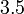$3.5$ if I purchase a loaf of bread every second day.
• Individual demand curves are more likely to exhibit sharp discontinuities for other reasons: Individuals may use threshold prices and reference prices to determine which item to purchase and how much. For instance, if, for me,$A$ and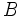$B$ are equivalent goods (i.e., they are perfect substitute goods for each other), then I buy none of$A$ when its price exceeds that of$B$, but I shift my entire consumption to$A$ when its price drops below that of$B$. The price of$B$ is thus a point of discontinuity in the demand curve for$A$. In the aggregate, the heterogeneity of individuals ensures that they do not all perceive the same pairs of goods as perfect substitutes, and hence these jumps are less likely to occur.
• Various violations of the law of demand (the assertion that demand curves slope downwards), both rational and irrational, are more likely to be seen at the individual level than at the aggregate level: For instance, the Giffen good phenomenon and the Veblen good phenomenon may play an important role in the consumption behavior of one individual or household, but because of differing incomes and differing tastes and preferences that lead individuals to value substitutes differently, the phenomena would not apply to all economic actors. Since an aggregate Giffen good phenomenon depends on the phenomenon affecting a large number of individuals, aggregate Giffen good phenomena may be much rarer than individual Giffen good phenomena. The same holds for various forms of mild irrationality and idiosyncratic behavior.

## Examples of demand curves

This gives some mathematical possibilities for the demand curve. The list is illustrative and not intended to be exhaustive.

### Demand curve for fixed total budget: reciprocal relationship between price and quantity

Here, the individual or household spends a fixed amount of money on the commodity regardless of its price. Thus, the quantity purchased is inversely proportional to the unit price, i.e., the demand curve equation is given by: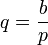$\! q = \frac{b}{p}$

where$b$ is the total budget amount that the household spends. Here are some properties of such a demand curve:

• The price-elasticity of demand for this demand curve is$-1$.
• As the price falls to$0$, quantity demanded goes to infinity. An actual demand of$\infty$ (infinity) may be infeasible, hence the above expression may not work for very low prices. However, such a demand curve may well explain what appears to be a zero price effect.
• For the quantity demanded to become zero, the price needs to be raised to infinity. Again, this may not be the case in reality, since with a sufficiently high price, the quantity demanded may be so low that the transaction costs dominate the picture.

A slight variant of the above is: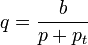$\! q = \frac{b}{p + p_t}$

where$p_t > 0$ is the transaction cost (which we assume as a cost per unit quantity purchased). This variant avoids the problem of infinities at one end: as the price falls to$0$, quantity demanded goes to$b/p_t$. In particular, for small transaction costs, this quantity demanded is extremely high.

Yet another variant involves two kinds of transaction costs: a transaction cost per unit, and a transaction cost that is constant for all nonzero amounts purchased and$0$ if nothing is purchased. If we denote these two transaction costs as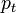$p_t$ (per unit) and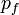$p_f$ (fixed) then: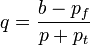$\! q = \frac{b - p_f}{p + p_t}$

Yet another variant is where there is a minimum quantity that can be demanded if any nonzero quantity is demanded, which we call$q_\min$. In this case, we first use the formula to determine quantity demanded. If the quantity demanded is less than$q_\min$, we replace it by$0$.

### Linear demand curve

Here, the demand curve is a straight line and is given by an equation of the form:$\! \frac{q}{q_0} + \frac{p}{p_0} = 1 \qquad \operatorname{for} \qquad p \le p_0, \qquad q = 0 \qquad \operatorname{for} \qquad p \ge p_0$

Here,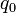$q_0$ is the quantity purchased at zero price and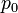$p_0$ is the minimum price needed for the quantity purchased to drop to$0$. Some features of this demand curve are:

• The magnitude of price-elasticity of demand is variable. It is less than$1$ for prices greater than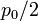$p_0/2$ and greater than$1$ for prices less than$p_0/2$. The total budget allocated is maximum for a price of$p_0/2$ and a quantity purchased of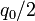$q_0/2$.
• The quantity purchased at a price of$0$ is$q_0$.
• The minimum price required to push the quantity purchased to$0$ is$p_0$.

### Demand curve with a region of high price elasticity in between

In the demand curve shown above, at a unit price of about 3, the demand curve is at its most elastic. For a very small variation in price around this point, demand goes from 2 units to 4 units. For prices greater than or less than 3, the price elasticity is considerably less. The high price elasticity at a price of 3 may arise because that is the price of a close substitute.

### Multiple demand curves

The picture given above shows two different demand curves for the same good. A change in some exogenous parameter that is one of the determinants of demand may lead to an expansion from the inner (blue) demand curve to the outer (purple) demand curve.

For a unit price of 2, the inner demand curve indicates a quantity of 1 unit demanded, while the outer demand curve indicates a quantity of 1 unit demanded.

Similarly, to achieve a quantity demanded of 3 units, a unit price of 2/3 is needed with the inner demand curve, but a unit price of 4/3 is needed with the outer demand curve.

## Curve characteristics

### Slope or first derivative

The slope (or rate of change) of the demand curve is inversely related of what is termed the price-elasticity of demand. The price-elasticity measures the total change in quantity demanded per unit change in price, made dimensionless by dividing by the quantity-price ratio. In other words, at a price$p$ and quantity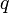$q$, the price-elasticity of demand is: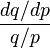$\! \frac{dq/dp}{q/p}$

The slope in mathematical jargon would be: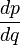$\! \frac{dp}{dq}$

### Sign of slope

Further information: Law of demand

Typically, the price-elasticity of demand is negative, which is equivalent to saying that the slope of the demand curve is negative, or the demand curve is downward-sloping. In other words, ceteris paribus:

• A decrease in the price per unit leads to an increase in the total quantity demanded.
• An increase in the price per unit leads to a decrease in the total quantity demanded.

This is termed the law of demand. There are two broad explanations for the law of demand:

A demand curve that violates the law of demand is termed an exceptional demand curve. One source of exception to the law of demand is Veblen goods, whose demand is related to conspicuous consumption. Other goods that appear to violate the law of demand (though they do not directly violate it) are Giffen goods and certain kinds of goods where there is inadequate information about quality and a higher price may be taken as a signal of higher quality.

### Sign of second derivative

There is no general principle that uniformly predicts the sign of the second derivative; similarly, there is no overarching rule about whether the price-elasticity of demand rises or falls with price.

In general, there are certain price ranges where the price-elasticity of demand is particularly high. These, in general, tend to be the price ranges where the substitution effect operates most strongly, i.e., the price ranges where there could be significant changes in the extent to which to substitute towards and away from substitutes. Alternatively, these are the price ranges where the reservation prices of many households are clustered.

In general below these price ranges, quantity demanded for the good is high, but largely price-inelastic, while above these price ranges, quantity demanded for the good is low, but again largely price-inelastic.

In this model of some critical price ranges, the sign of the second derivative is not constant.

In general, the behavior of the second derivative depends on the following:

• At the level of individual households, it depends on the second derivative of the marginal utility curve, or the third derivative of the utility curve.
• At the level of an economy, it depends on the distribution of income as well as of tastes and preferences in the economy.

## Empirical estimation

Further information: Demand curve estimation

The demand curve is difficult to estimate because at any given point in time, we can only get one (quantity, price) pair for the market as a whole. There are, however, ways around this problem that allow us to make a rough sketch of the demand curve.

## Effect of sales tax on demand curve

Further information: effect of sales tax on market price and quantity traded

For some goods, a sales tax is levied on the good, usually either a fixed amount per unit of the good sold, or as a fixed proportion of the pre-tax price. In case of a sales tax, there are two possible conventions used when drawing the demand curve:

• In one convention, the unit price refers to the pre-tax price. In this case, an increase in the sales tax leads to a contraction of the demand curve, and a decrease in the sales tax leads to an expansion of the demand curve.
• In the other convention, the unit price refers to the price inclusive of taxes. In this case, a change in the sales tax has no effect on the demand curve; it affects the supply curve instead.

## Movement along the curve

Further information: convergence towards market price

Any particular price corresponds to a point on the demand curve: the price coordinate of the point is that price, while the quantity coordinate is the quantity demanded at the price. Changes in price, while keeping other factors constant, is termed movement along the demand curve. Decreasing price with time is termed riding down the demand curve (also called price skimming) while increasing price with time is termed riding up the demand curve.

In a free market, suppliers are expected to vary their price until demand equals supply. In other words, demand moves along the demand curve until the price reaches the market price.

## Movement (shifts) of the curve

Further information: comparative statics for demand and supply

The demand curve changes when one (or more) of the determinants of demand other than price changes.

### Outward shift of the demand curve

An outward shift of the demand curve (also called an expansion of the demand curve) indicates an increase in the demand at every price, or equivalently, an increase in the price needed to restrict demand to a particular level.

### Inward shift of the demand curve

An inward shift of the demand curve (also called a contraction of the demand curve) indicates a decrease in the demand at every price, or equivalently, a decrease in the price needed to restrict demand to a particular level.

### Other shape changes of the demand curve

The demand curve may change in shape in a way that moves it inward for some price levels and outward for other price levels. This may happen in the case of the individual demand curve if the degree of substitution with another substitute good increases. It may happen for the market demand curve due to a change in the income distribution.